Google Spreadsheet software is a great tool where we can create magic with our datasets. We use various formulas and functions to structure, organize, and perform mathematical operations in Google Sheets. But, when we are using functions or formulas in Google Sheets, we just the result not the formula or function used. However, the spreadsheet also allows us to see what formula or function is used in a particular cell with the help of its in-built feature.

## How to Show Formulas Instead of Values in Entire Google Sheets?

Let’s consider the following dataset. Here I have various formulas in each cell of the spreadsheet.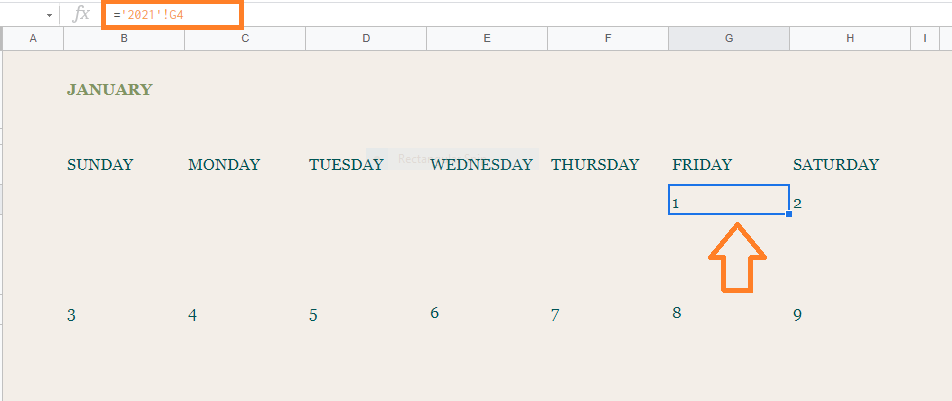Now follow the steps as listed below to show formulas instead of values:

• Step 1: Open the Google Spreadsheet where you would like to show formulas instead of values.
• Step 2: Click on the “View” tab in the menubar.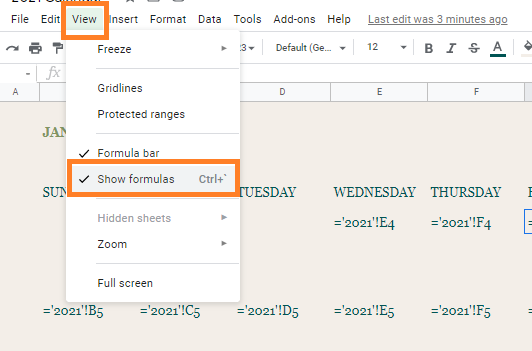• Step 3: Now select “Show Formulas” from the drop-down menu.
• Step 4: This will automatically show formulas instead of values in Google Sheets.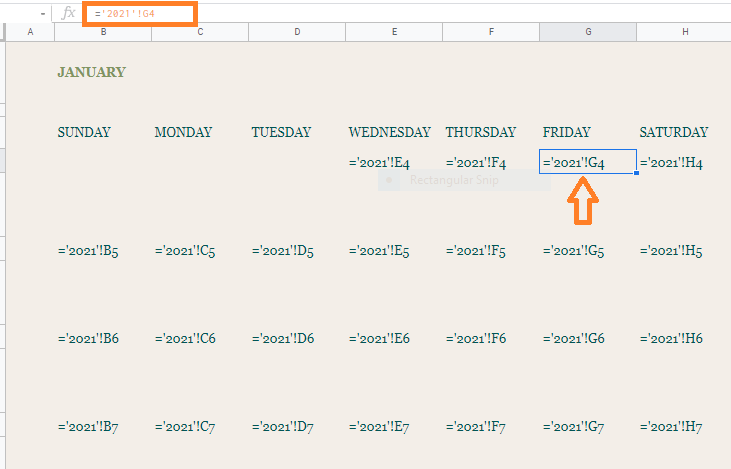This strategy can help you grasp what’s going on in a spreadsheet that you are unfamiliar with. Looking at someone else’s work is also a great approach to learn how to use formulas and analysis tools.

This view is very useful when analyzing a workbook that appears to be returning incorrect values. This allows you to see all of the formulas at once rather than having to click on each cell to figure out what’s going on.

In order to convert back formulas to values follow the steps as listed below:

• Step 2: Now uncheck “Show Formulas” from the drop-down menu.

This will show values now instead of formulas.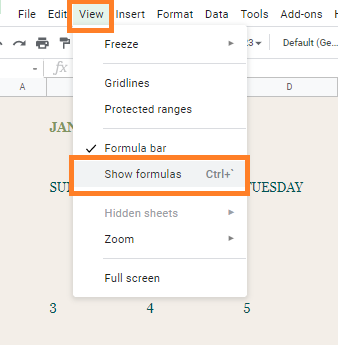### How to Show both Formula and Values at the Same Time?

In Google Sheets, we can see both formulas and values at the same time. To know how to do this, follow the steps as listed below:

• Step 1: Select the cell where you would like to view the formula.
• Step 2: Double click the cell to see the detailed functions of the cell.
• Step 3: Click on the “?” icon next to the cell to bring up the pop-up value preview. Alternatively, you can also “F9” key to enable the pop-up value preview.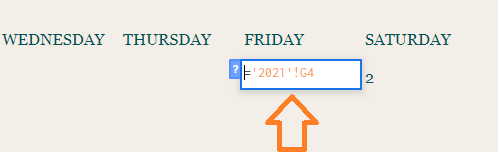These tricks come in handy when trying to understand what’s going on with a particular formula in the cell. So you can fix formulas and see all the information at once.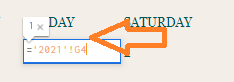### How to Show Formula by Converting it to Text?

This method can be adopted if you want the values to display as formulas permanently.

In order to compel Sheets to show instead than process a formula, you only need to place the formula in apostrophe before it.

Google Sheets doesn’t include a right-click menu or other formatting choices to control cell formatting, unlike other cell formatting approaches. Changing the formula in the cell is the only option.

Now follow the steps listed below to show formulas by converting them to text.

• Step 1: Select the cell where you would like to show formulas instead of values.
• Step 2: Now simply add an apostrophe before the formula.
• Step 3: Press the “Enter” button. The apostrophe will be hidden in Google Sheets and the only formula will be displayed as shown below.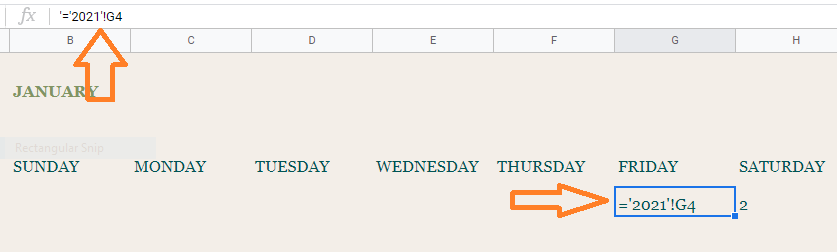Your formula will be displayed instead of the value when the apostrophe symbol is used. Apostrophes in one cell will replace all cell contents with that cell’s value if you try to move the value across a range. When you add an apostrophe to a calculation, Sheets will not automatically modify the formulas.

### How to Show formulas instead of values in Google Sheets using Keyboard Shortcut?

This is one of the easiest methods where you don’t have to execute multiple steps. The steps to achieve “shows formulas instead of values” are given below:

• Step 2: Now simply use the following keys on your keyboard ” Control,” and “Accent“. ( Ctrl + `)
• Step 3: Press all the keys in one go. This will show formulas now instead of values in Google Sheets.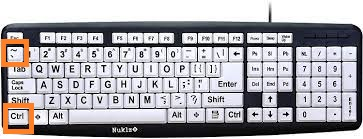### Shows Formulas instead of Values by Clicking on Cell

This is one of the normal methods where you can simply show formulas instead of values. Just double click on the cell and the Google Sheets will show formulas instead of values. The limitation of this method is that we have to execute this step at each and every cell.## How to use REGEXEXTRACT Function in Google Sheets?(With Examples)

REGEXEXTRACT function in google sheets extracts the first matching substrings according to a regular expression. Regular expressions are sophisticated search patterns or character sequences that allow you to find specific patterns in a string. Once found, they can be replaced with any other text using the REGEXEXTRACT function.

The REGEXEXTRACT is one of the three regex functions in google sheets along with the REGEXREPLACE and REGEXMATCH functions.

## What Does the REGEXEXTRACT Function in Google Sheets Do?

The REGEXEXTRACT function in google sheets extracts the first matching substrings according to a regular expression. The REGEX function is called a regular function that matches a particular pattern and replaces it with different text.

## Syntax of the REGEXEXTRACT Function in Google Sheets

=REGEXEXTRACT(text, reg_exp)

In the above syntax,

• text – is the text or string that has to be replaced
• reg_exp – is the regular expression with a particular pattern. This expression matches part of the text or string that has to be replaced. The regular expression parameter should be provided in double-quotes

## Application of the REGEXEXTRACT Function in Google Sheets

When you want to extract a set of strings that are not exactly similar or consistent in format, the REGEXEXTRACT function can be quite useful.

Below are some of the useful applications of the REGEXEXTRACT function in google sheets,

• It can be used to extract the first or last few characters from a string
• It can be used to extract numbers from a string
• It can be used to extract whole words based on a partial match
• It can be used to extract one of a list of words
• It can be used to extract contents between certain characters
• It can be used to extract different parts of a URL
• It can be used to extract different parts of email addresses

There are many other ways also in which you can use the REGEXEXTRACT function in Google sheets.

Let’s see in detail how the REGEXEXTRACT function is used in the above application below,

### Using the REGEXEXTRACT Function in Google Sheets to Extract the First or Last few Characters from a String

Here let’s see how to extract the first or last few characters in a string using the REGEXEXTRACT function in google sheets from the selected cell,

Extract the First few Characters from a String:

To extract the first few characters from a string we can use the REGEXEXTRACT function in the google sheets.

The below formula can be used to extract the first few characters,

=REGEXEXTRACT(text,”…”)

In the above expression, the three dots represent “…” the first three characters. If you need to extract only the first two characters then you could give two dots in double quotes “..”

Example,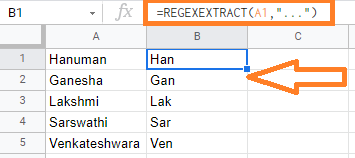In the above example, we are extracting the first three characters from the string with the expression, =REGEXEXTRACT(A1, “…”)

Extract the Last few Characters from a String:

To extract the last few characters from a string we can use the REGEXEXTRACT function in the google sheets.

The below formula can be used to extract the last few characters,

=REGEXEXTRACT(text,”…\$”)

In the above expression, the dollar(\$) symbol is suffixed to the dots. above expression represent “…\$” the last three characters. If you need to extract only the last two characters then you could give two dots ending with dollar symbol in double quotes “..\$”

Example,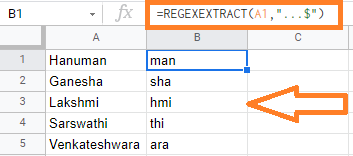In the above example, we are extracting the last three characters from the string with the expression, =REGEXEXTRACT(A1, “…\$”)

Extract the first word from a String:

Additionally, to extract only alphanumeric characters, then you need to use \w metacharacter which represents a single alphanumeric character (a digit, a letter, or an underscore) instead of the dot symbols.

Suppose if you have the first name and last name and you need to extract the first name alone, then you could use below expression,

=REGEXEXTRACT(A1,”\w+”)

In the above expression, “\w+” is used to extract the first word in the given text.

Example,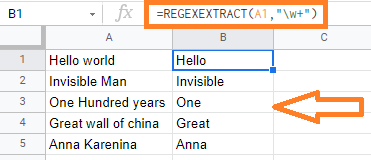In the above example, we are extracting the first word with the expression, =REGEXEXTRACT(A1, “\w+”)

Extract the last word from a String:

To extract the last name or the word then you could use the below expression,

=REGEXEXTRACT(A1, “\w+\$”)

In the above expression, “\w+\$” is used to extract the last word in the given text. We are suffixing with the dollar symbol (\$)

Example,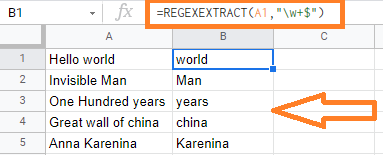In the above example, we are extracting the last word with the expression, =REGEXEXTRACT(A1, “\w+\$”)

### Using the REGEXEXTRACT Function in Google Sheets to Extract Numbers from a String

Here let’s see how to extract the numbers in a string using the REGEXEXTRACT function in google sheets from the selected cell.

Extract the first numbers from a string:

To extract the first numbers from the String, we can use the expression “\d+”. The \d metacharacter represents the numeric digit.

Below expression can be used to extract the first numbers in the string,

=REGEXEXTRACT(text, “\d+”)

Example,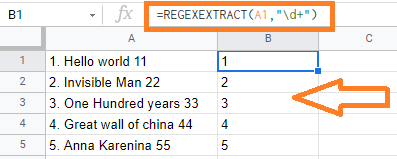In the above example, the first numbers are extracted with the expression, =REGEXEXTRACT(A1, “\d+”)

Extract the last numbers from a string:

To extract the last numbers from the String, we can use the expression “\d+\$”. The \d metacharacter represents the numeric digit. The dollar symbol (\$) is suffixed to extract the last numbers

Below expression can be used to extract the last numbers in the string,

=REGEXEXTRACT(text, “\d+\$”)

Example,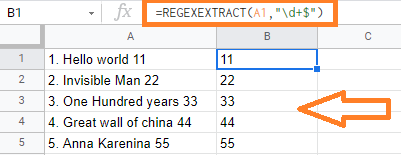In the above example, the last numbers are extracted with the expression, =REGEXEXTRACT(A1, “\d+\$”)

### Using the REGEXEXTRACT Function in Google Sheets to Extract Whole words based on Partial Match

Here let’s see how to extract whole words based on Partial Match in a string using the REGEXEXTRACT function in google sheets from the selected cell.

Example:

To extract the word which is matching the starting letter ‘bo’ and ending with the letter ‘d’, we use the below expression,

=REGEXEXTRACT(A1,”bo\w+d”)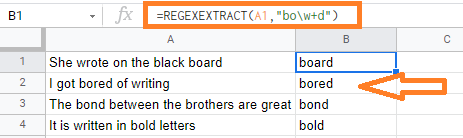In the above example, you could see that the string that staring with the letter “bo” and ending with “d” are extracted.

### Using the REGEXEXTARCT Function in Google Sheets to Extract One of a List of Words

Here let’s see how to extract one of a list of words in a string using the REGEXEXTRACT function in google sheets from the selected cell.

The or operation is represented by the metacharacter ‘|’. If you want to extract one word from a collection of words or characters, you can use the REGEXMATCH function in Google Sheets.

Example: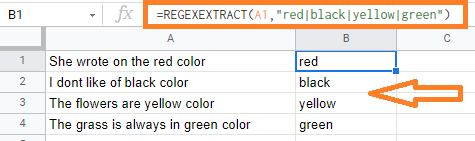In the above example, we are extracting few words i.e., red, black, yellow, and green in all the selected cells with the expression =REGEXEXTRACT(A1, “red|black|yellow|green”)

### Using the REGEXEXTRACT Function in Google Sheets to Extract Contents between Certain Characters

Here let’s see how to extract contents between certain characters using the REGEXEXTRACT function in google sheets from the selected cell.

Example: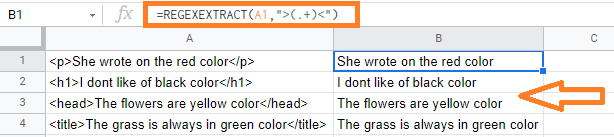In the above example, we are trying to remove the HTML tags with the expression          =REGEXEXTRACT(A1, “>(.+)<“). Here we are searching for the first symbol starting with “>” and the first symbol starting with “<“. So the text in between the above-specified symbols is returned.

### Using the REGEXEXTRACT Function in Google Sheets to Extract Different Parts of a URL

Here let’s see how to extract different parts of a URL in the string using the REGEXEXTRACT function in google sheets from the selected cell.

The below expression can be used to extract the domain name from the URL,

=REGEXEXTRACT(A1,”http.+\ / \ /(.+)\ /”)

The above expression will extract all the contents in between the pattern “HTTP://” and the “/” symbol

Example: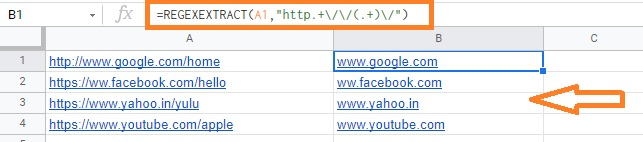In the above example, you could see we have extracted only the email domain name.

### Using the REGEXEXTRACT Function in Google Sheets to Extract Different Parts of an Email Address

Here let’s see how to extract different parts of an Email Address using the REGEXEXTRACT function in google sheets from the selected cell.

To extract only the username in the email address you can use the below expression,

=REGEXEXTRACT(A1,”(.+)@”)

Example: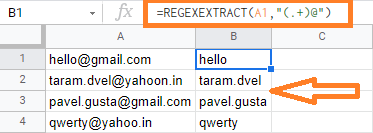In the above example, we have extracted only the username in the email address.

Have you ever been taught about creating yearly or monthly Calendars in Google Sheets? If not, here is an article, where you will learn about how to create a calendar template with the help of Google Sheet tips provided on this page.

• Step 2: Now move to the Google Apps Section and click on it.
• Step 3: Select “Sheets” from the drop down menu.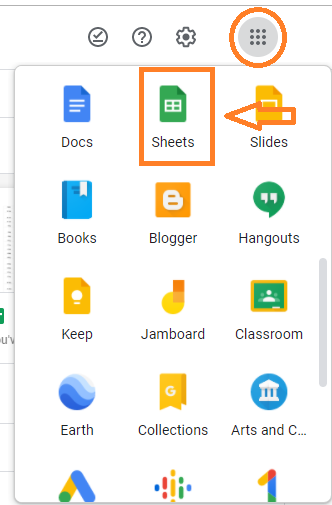• Step 4: Now in the Google Sheets window, click on the “Template Gallery“.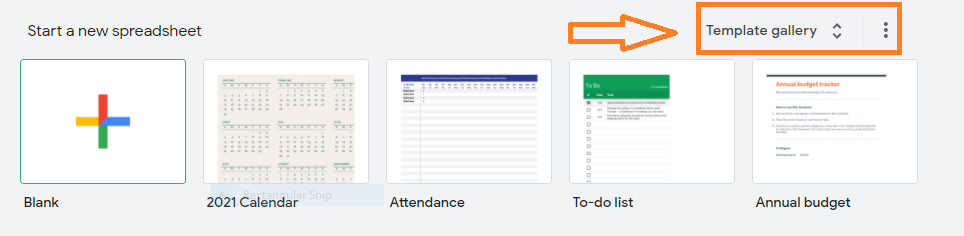• Step 5: Under the “Personal” window, you will find the year-wise calendars. Click on “2021 calendar” for the 2021 calendar.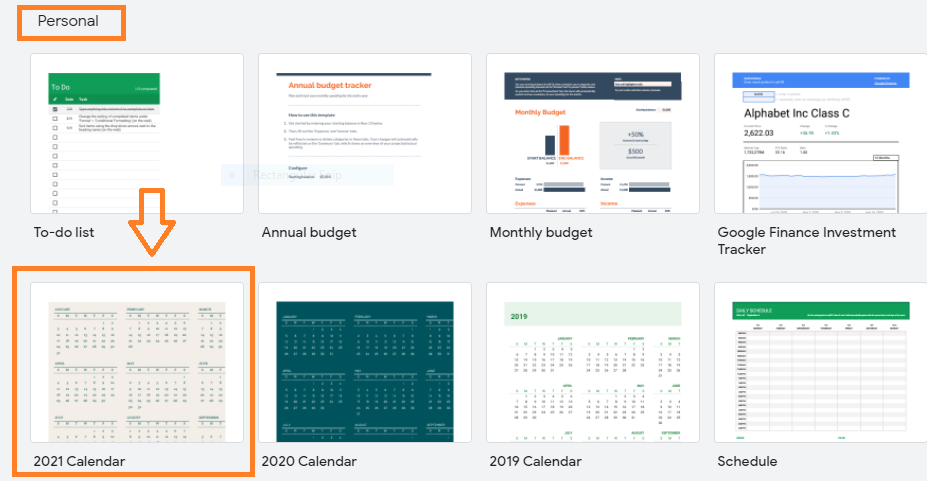• Step 6: The calendar template sheet will open on your device.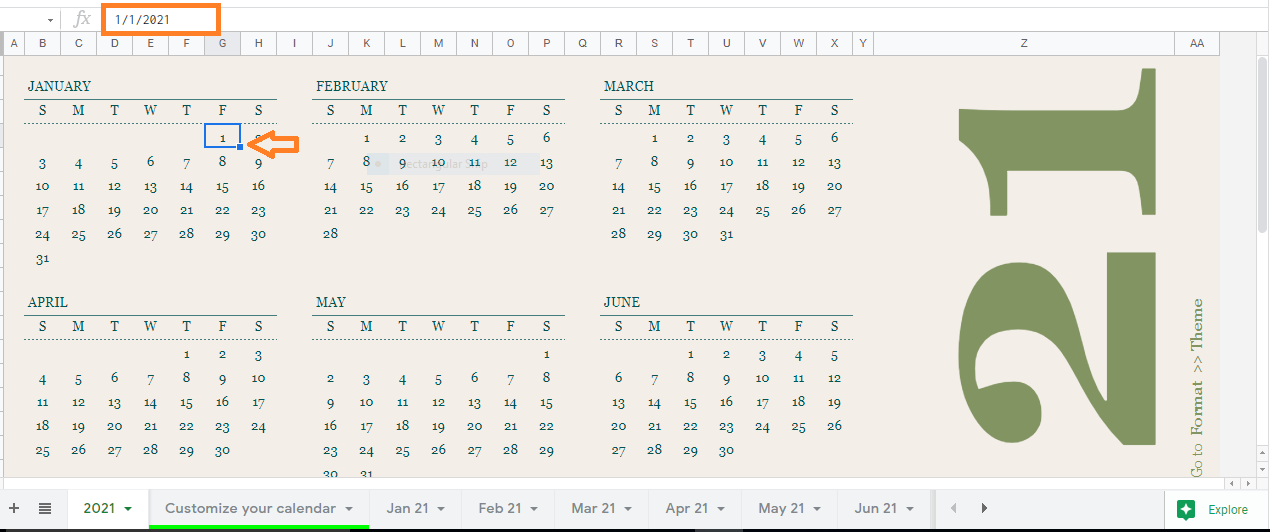### How to Customize the Google Sheets Calendar Template?

In this article, I have chosen the 2021 inbuilt calendar template. Once the 2021 calendar template is opened on your screen, move to the “Customize your calendar” tab. Here you will have 16 different themes to customize your calendar for 2021.

The steps to customize the 2021 calendar is as follows:

• Step 1: Simply select “Theme” from the “Format” menu.
• Step 2: In the side panel, browse and select one of the preset Themes.
• Step 3: You can use the themes as is, or you can customize them by clicking “Customize.
• Step 4: Choose the theme which you require and start customizing the Google Sheets calendar template.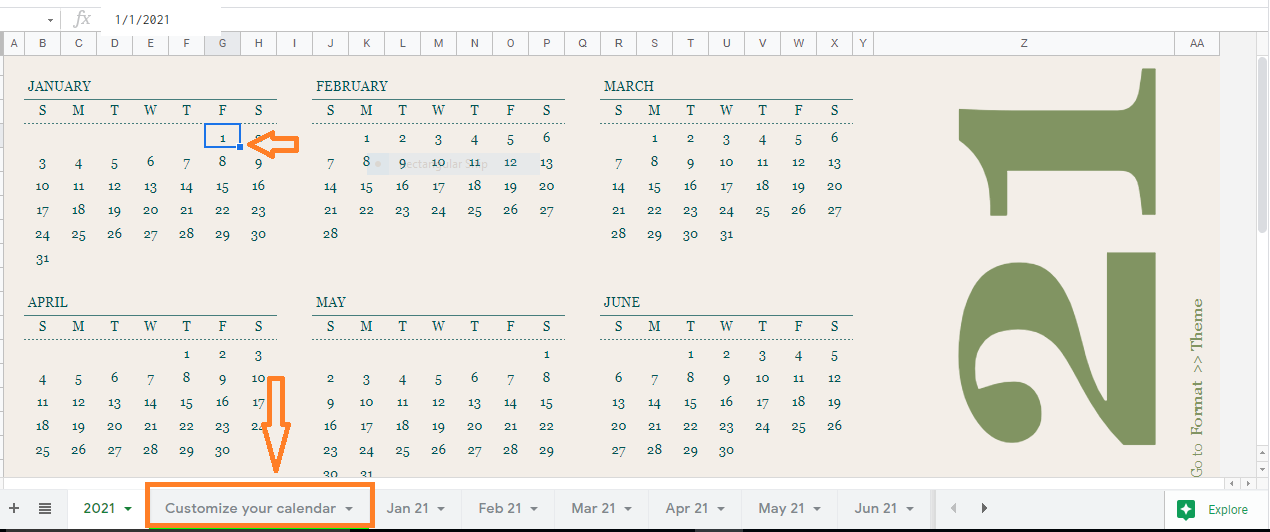### Explanation of Google Sheets Calendar Template

Firstly, a sheet tab named 2021 has been created in Google Spreadsheet. In this sheet, the 2021 yearly dataset has been added such as the list of months, dates as per the day, and so on.You can easily replicate this yearly calendar template. All you have to do is, add 12 months and move to the start day of January 2021. Now simply add the date manually and used the fill handle to fill upcoming dates. By doing so you can format the yearly calendar in less than 10 minutes.

Once the yearly calendar has been created, 12 different sheet tabs have been added to the 2021 calendar sheet. Each tab is named with a month name such as Jan 2021, Feb 2021, and so on.

Now move to the Jan 2021 sheet tab. Here enter 7 days’ names in the week such as Monday, Tuesday, and so on. The year 2021 commenced on a weekday Friday. So move to the Friday Cell and simply enter the formula “=‘2021’!G4″.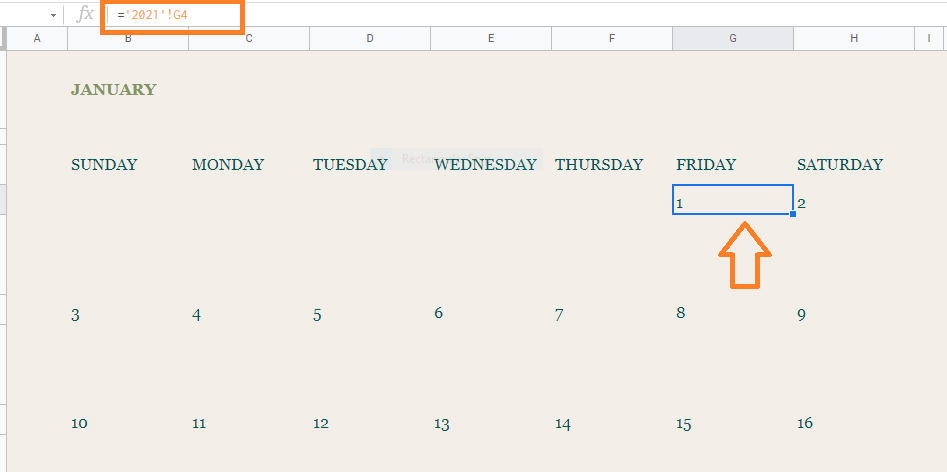Formula Explanation =‘2021’! refers to the sheet name. 2021 is the yearly calendar tab that we created earlier. So basically we are calling the value from the cell G4 in the 2021 sheet tab. Since cell G4 has the value as “1”, it is reflected here. Now use the fill handle to apply the formulas to other cells which will fetch the dates from the yearly calendar (2021) to update the dates in the monthly calendar.

Follow the above process for the next 11 months and Google Sheets yearly and monthly calendar template is ready.

## How to Refresh Pivot Table in Google Sheets: 3 Ways to Refresh Pivot Tables

Google Sheets Pivot Table is a dynamic approach to summarise vast volumes of data rapidly. A Pivot Table can be used to study numerical data in depth and to answer unexpected queries about your data. However in some instances, if even there is a modification in data, the Pivot table wouldn’t show up. This is where we need to refresh the Pivot table.

## How to Refresh Pivot Table in Google Sheet?

Pivot tables don’t need to be refreshed manually in most cases. Whenever the data being pulled by the table is updated, they are automatically refreshed to reflect the new information. As a result, you may still encounter circumstances where the Pivot table data is not updated.

### Why Pivot Tables doesn’t Refresh?

The pivot table doesn’t refresh because of the following reasons:

• If you add data outside the Pivot table range.
• If you use filters in the Pivot table
• If your dataset contains formulas that need to be re-calculated.

### Pivot Table Doesn’t Refresh While Adding New Row

A pivot table is created based on the cell range specified when generating the table. Suppose if you add a row or column outside the Pivot table cell range, the table will not reflect the data.

Solution:

There are many instances where we would like to add more rows, once the pivot table is generated. In order to avoid this kind of issue, while creating a pivot table, just select more cell ranges.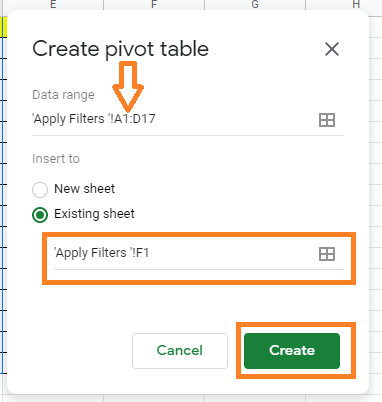### Pivot Table Doesn’t Refresh if Filters are Used

If your pivot table consists of filters, then your data will not be updated, even you make changes in the original data sets. If the Pivot table employs filters, your data will not be updated when the original data values change. This is a kind of restriction in the Google Sheets Pivot table.

Solution:

The only solution to overcome this problem is to remove the filters from the Pivot table.

Remove the filters in the Google Sheets Pivot table and then make the necessary changes in the original data. Once the necessary changes are made, click on the “Filters” category under the Pivot table editor and apply the filters. Now you will see the necessary changes.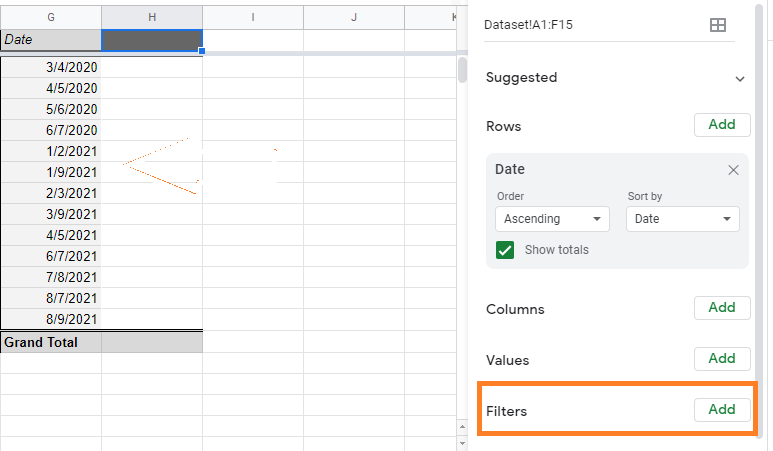### Pivot Table Doesn’t Refresh When Formula Needs To Re-Calculated

The Pivot table doesn’t refresh when we use the formulas such as RANDOM and TODAY. This is because the pivot table doesn’t work well when we use the functions which need to refresh.

Unfortunately, there is no solution for this problem, all we have to do is avoid this kind of function when creating the Pivot table in Google Sheets.

## How to use REGEXREPLACE Function in Google Sheets? (With Examples)

REGEXREPLACE function in google sheets is used to replace a part of a text string with a different text string using regular expression.

Regular expressions are sophisticated search patterns or character sequences that allow you to find specific patterns in a string. Once found, they can be replaced with any other text using the REGEXREPLACE function.

The REGEXREPLACE is one of the three regex functions in google sheets along with the REGEXEXTRACT and REGEXMATCH functions.

## What Does the REGEXREPLACE Function in Google Sheets Do?

The REGEXREPLACE function in google sheets simply replaces the part of the text string with a different text string. The REGEX function is called a regular function which matches a particular pattern and replaces it with different text.

## Syntax of the REGEXREPLACE Function in Google Sheets

=REGEXMATCH(text, reg_exp, replace_text)

In the above syntax,

• text – is the text or string that has to be replaced
• reg_exp – is the regular expression with a particular pattern. This expression matches part of text or string that has to be replaced
• replace_text – is the text that will be replaced with all the parts of text or string that match the reg_exp

### Application of the REGEXREPLACE Function in Google Sheets

When you want to replace a specific search string or pattern in multiple cells, the REGEXREPLACE function in google sheets can be very useful.

Below are some of the useful applications of the REGEXREPLACE function in google sheets,

• It can be used to replace or remove a specific letter, word, or phrase in different cells.
• It can be used to remove or replace all spaces in a text.
• It can be used to delete or replace all numerical values in a string.
• It can be used to remove or replace all URLs in a string.
• It can be used to remove HTML tags from a string.

There are many other ways also in which you can use the REGEXREPLACE function in Google sheets.

Let’s see in detail how the REGEXREPLACE function is used in the above application below,

### Using REGEXREPLACE Function in Goole Sheets to Replace or Remove a Letter, Word, or Phrase in a String

Here let’s see how to remove a specific letter, word, or phrase in a string using the REGEXREPLACE function in google sheets from the selected cell,

Example,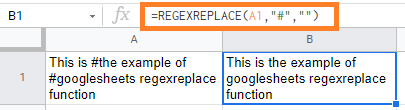In the above example, If we want to remove the hash symbol ‘#’ from the text in the A1 cell.

For this, we are using the REGEXREPLACE function as follows,

=REGEXREPLACE(A1, “#”, “”)

Here we are replacing the ‘#’ with nothing.

If we want to replace the string “example” with “sample” then we can use below REGEXREPLACE function,

=REGEXREPLACE(A1, “example”, “sample”)

Below is the result you would get,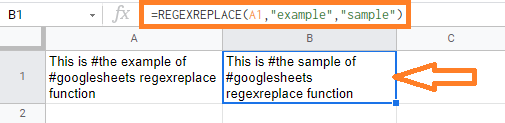We can also use the REGEXREPLACE function for removing and replacing more than one word in the cell.

Example: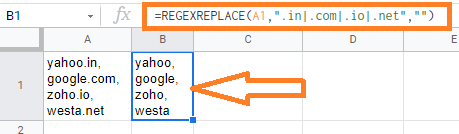In the above example,

We are removing multiple words like “.in, .com, .io, .net” from yahoo.in, google.com, zoho.in, westa.net with below formula,

=REGEXREPLACE(A1, “.in|.com|.io|.net”, “”)

The above expression is converted to Yahoo, Google, Zoho, Westa.

### Using REGEXREPLACE Function in Google Sheets to Remove All the Spaces From a String

Here let’s see how to remove the spaces in a string using the REGEXREPLACE function in google sheets from the selected cell,

Example,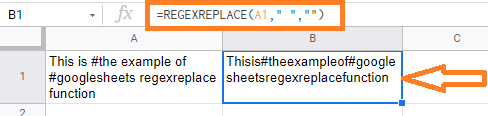In the above example, we are replacing the spaces with no space with the below expression,

=REGEXREPLACE(A1, ” “, “‘)

This expression removes all the spaces from the A1 cell text.

We could also replace the space with special characters like (!, @, #, \$, and so on) and also with any of the alphabets.

Example: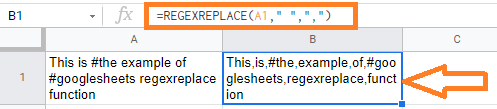In the above example,

=REGEXREPLACE(A1, ” “, “,”)

We are replacing the empty spaces with a comma – “,” in A1 cell text in the above expression.

### Using the REGEXREPLACE Function in Google Sheets to Remove or Replace All Numerical Values in a String

To replace or remove all the numbers from a cell, you need to use the “[0-9]” regex characters.

The square brackets are used to hold a set of characters. Since you want to match any number between 0 and 9, we use the 0-9 regular expression inside the square brackets.

This means ‘match any character that is between 0 and 9’.

Example: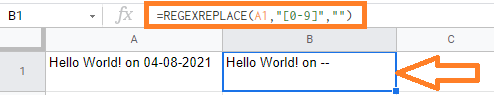In the above example,

=REGEXREPLACE(A1, “[0-9]”, “”)

We are removing the numbers from the A1 cell in the above expression.

Here, we can also remove or replace all the numbers which include decimal points.

Formula : =REGEXREPLACE(A1, “[0-9]*\.[0-9]|[0-9]”, “”)

In the above formula, we are using the “.” character preceded by the escape character “\” so that the “.” character is not mistaken for a regular expression.

The ‘*’ character denotes zero or more occurrences of a character or string, whereas the ‘+’ character denotes one or more occurrences of a character or string.

This ensures that the regular expression matches even numbers with no digits preceding the decimal point.

We added another [0-9] expression after the ‘|’ operator because we also want to consider cases where the number is an integer (with no decimal point at all).

Example: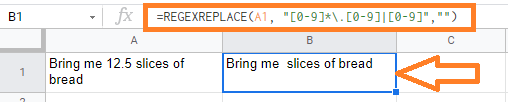In the above example, we are removing the decimal number 12.5.

### Using REGEXREPLACE function in Google Sheets to Remove or Replace Web URLs from the String

Here let’s see how to remove or replace the Web URLs in a string using the REGEXREPLACE function in google sheets from the selected cell.

To remove all the instances of web URL from the selected cell, we can use the below expression’s,

Formula:

• =REGEXREPLACE(A1, “www(.*)com”,””)
• =REGEXREPLACE(A1, “^www\.[a-zA-Z\.]+com”, “”)

This expression can be used to represent any number of characters. When we put this expression between the words ‘www’ and ‘com,’ it represents any string that begins with www and ends with com.

Example: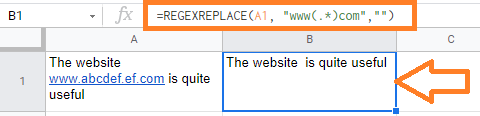In the above example, we are replacing the text prefixing “www” and ending with “com”.

### Using the REGEXREPLACE Function in Google Sheets to Remove HTML tags from a String

Here let’s see how to remove the HTML tags in a string using the REGEXREPLACE function in google sheets from the selected cell.

We can use the below formula to remove the HTML tags,

Formula:

=REGEXREPLACE(A1, “(\<([A-Za-z1-9]+)\>)|(\</([A-Za-z1-9]+)\>)”, “”)

The regular expression in the above formula can be divided into two parts,

• Opening tags – like <p> and <code>
• In the above formula , we are using the regular expression: (\<([A-Za-z1-9]+)\>). This matches all the instances starting with “<” and ends with “>”
• Closing tags – like </p> and </code>
• In the above formula , we are using the regular expression: (\</([A-Za-z1-9]+)\>). This matches all the instances starting with “</” and ends with “>”

The two expressions are separated with the ‘|’ symbol so that it matches either of the two sub-expression.

Example: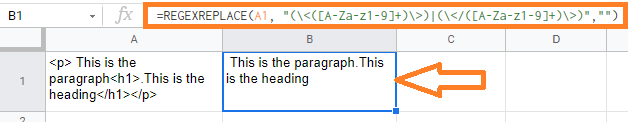In the above example, we are removing the HTML tags <p>,<h1>, </p>, </h1> in the A1 cell.

## How to Group by Month in Pivot Table in Google Sheets (With Examples)

A Pivot Table in Google Sheets is a function for summarising, sorting, reorganizing, grouping, counting, totaling, or averaging data in a table. It allows us to change rows into columns and columns into rows. It lets you organize your data by any field (column) and perform advanced calculations on it.

Also with the help of the Google Sheets Pivot table, we can group the data by week, month, year, and even a day. In this article, let’s understand how to group Pivot tables with the help of Google Sheet tips provided on this page. Read on to find out more.

## How to Group by Month in Google Sheets Pivot Table?

In order to group by month in the Google Sheets Pivot table, make sure your dataset is having the data values which are mentioned in proper date format.

Now let’s consider the following example where we can group by month in the Google Sheets Pivot table.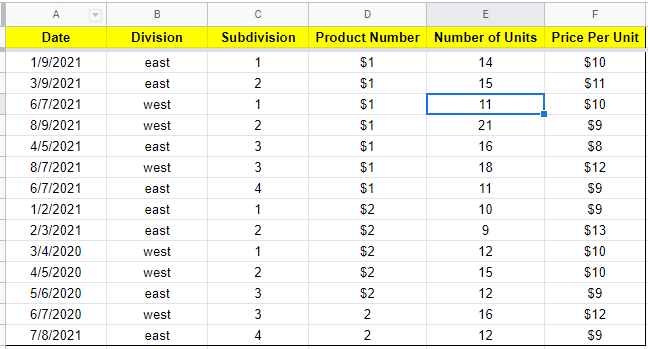### How to Create Date Values In Google Sheet?

Suppose if your dataset doesn’t have proper date values, then you can use the following steps to create the proper DATE format in Google Sheets.

• Step 1: Select the Date column, where you can create a proper DATE format.
• Step 2: Now click on the “Format” from the menubar and select “Number” from the drop-down menu.
• Step 3: The Number sub menu will be displayed on the screen. Choose “Date” from the sub-menu.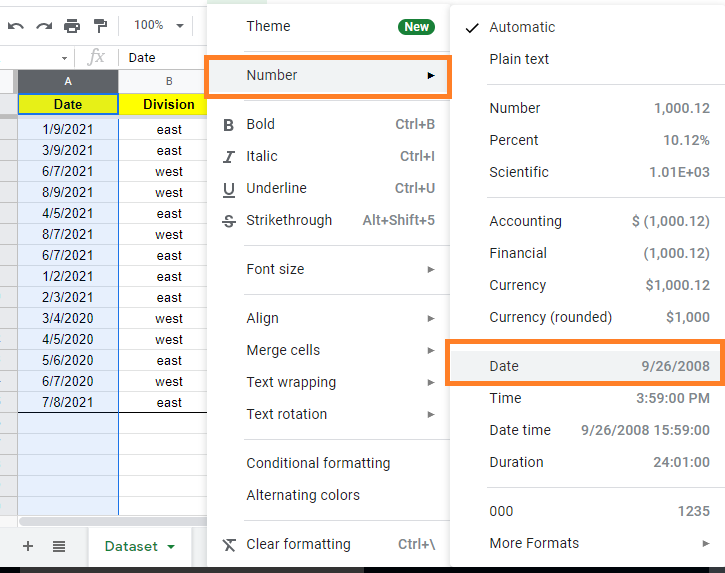The selected columns will now have Date Values in the form of Google Sheets DATE format.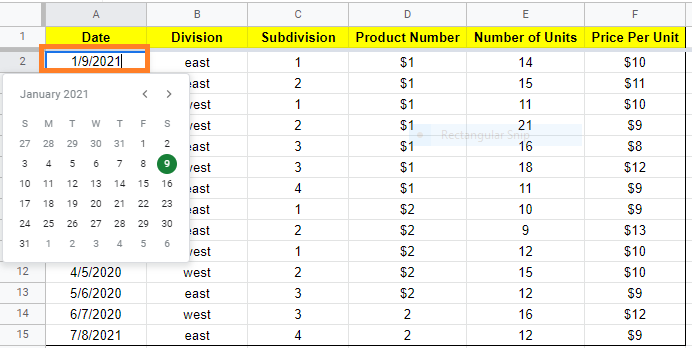### How to Create Pivot Table to Group Date-wise?

Consider the following dataset. Now follow the steps listed below to create a pivot table and group the date-wise.

• Step 1: Open the Google Sheet.
• Step 2: Click on the “Data” from the menubar and select “Pivot Table” from the drop-down menu.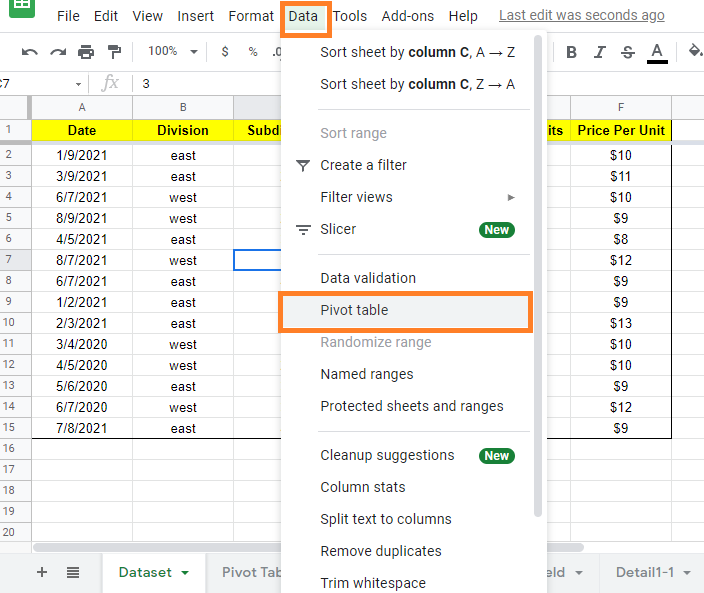• Step 3: Now the Pivot table box will appear on the screen. Here choose the data range and check the box where you would like to create the pivot table.
• Step 4: Once the necessary actions are taken, just click on the “Create” button.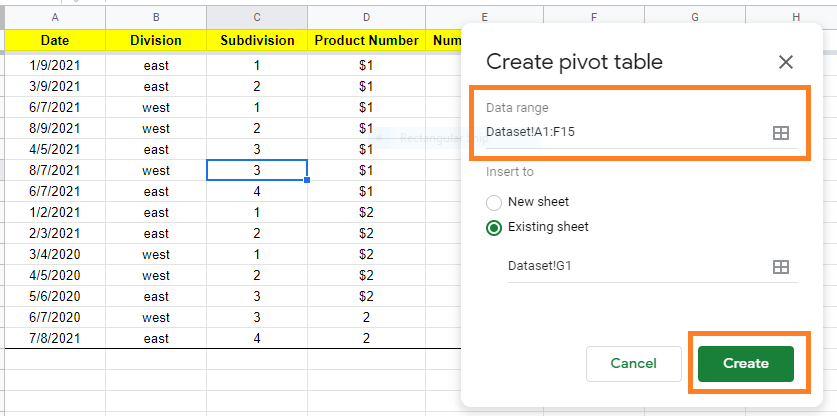• Step 5: This will create the Pivot table in the specified place. Now click on the Pivot table. The Pivot table editor will open on the screen.
• Step 6: Under the Pivot table editor, start adding your data by clicking the “Add” button under column, row, filter, values.
• Step 7: As soon you add the data, click on the “Add” button and choose “Date“.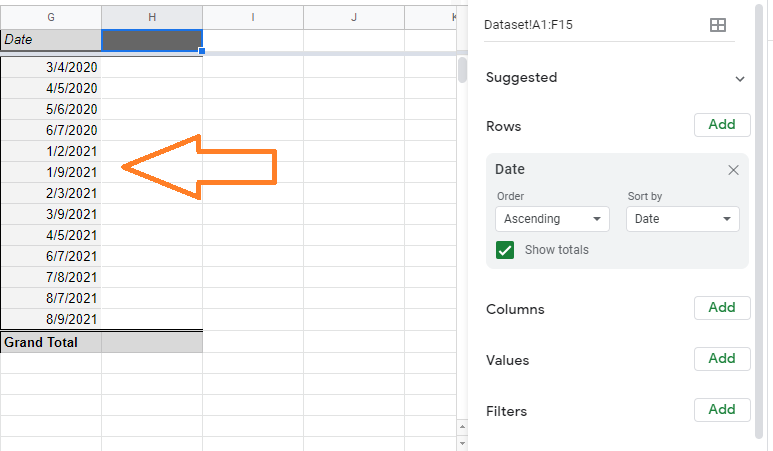This will order the data date-wise as shown below.

### Grouping Google Sheets Pivot Tables by Month

Follow the steps listed below to group the Google Sheets pivot tables by month.

• Step 1: In the previous section, we grouped the Pivot table date-wise.
• Step 2: Now right-click on any of the dates under the “Date” column.
• Step 3: The context menu will open up on the screen. Now select “Create Pivot table date group” from the context menu.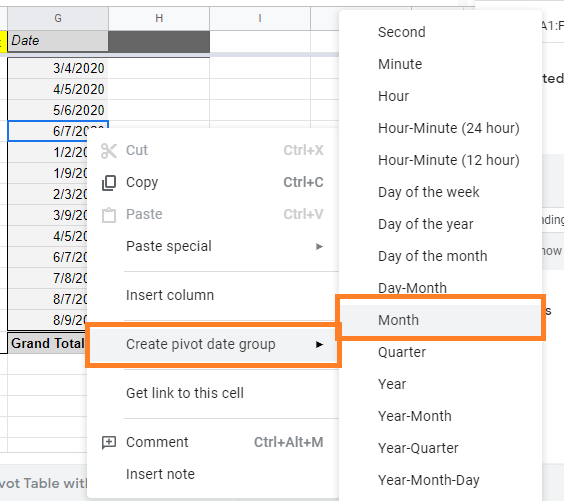• Step 4: Now another sub-menu will open on the screen. Here you will be provided with so many options. Choose “Month” in this sub-menu.
• Step 5: This will group the columns month-wise as shown below.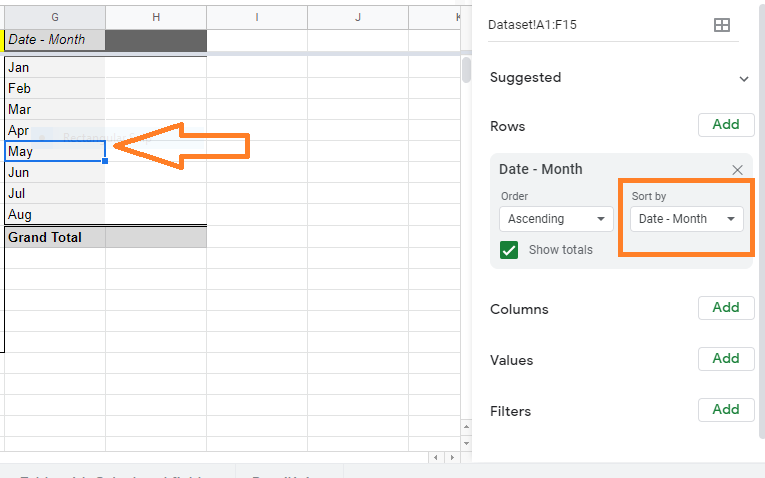To group Pivot Table by Year, right-click on the Date column and select “Year” from the context menu.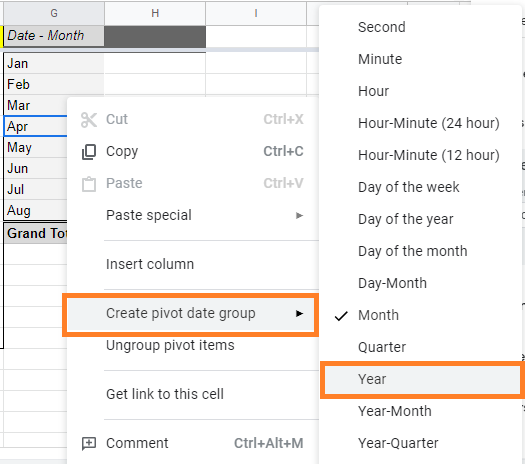When you group pivot table by year, the result will be as follows: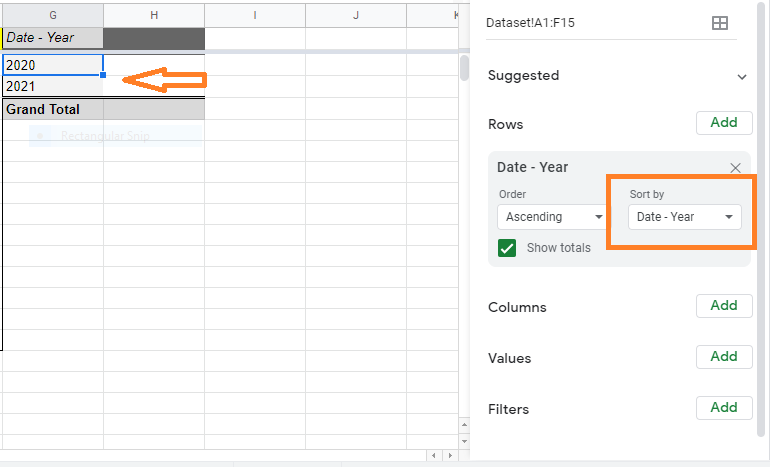## How to Round Numbers in Google Sheets? (ROUNDUP/ROUNDDOWN)

The rounding function in Google sheets will convert the decimal number to the nearest whole number, the second decimal point, hundreds, and by the factor of three. Google Sheets makes it easy to round the numbers with various rounding functions like ROUND, ROUNDUP, ROUNDDOWN, MROUND, and so on. In this article, let’s learn everything about Round Function with the help of Google Sheet tips provided on this page.

## ROUND Function in Google Sheets

The ROUND function can reduce or increase any value by a specific number of decimal places. The rounding digit will be rounded up or rounded down.

### Rounding Number to the Nearest Integer Number in Google Sheets

To round any decimal value to the nearest numeric value(integer) we can use the ROUND function in Google sheets.

• If the number to the right of the rounding digit is less than five, the rounding digit is not changed.
• If the number to the right of the rounding digit has a value of five or higher, the rounding digit is raised by one.

Below is the syntax for the ROUND function in google sheets,

=ROUND(value)

value – we should specify the decimal number which has to be round.

Example,

• =ROUND(2.6) – This will give you 3
• =ROUND(2.4) – This will give you 2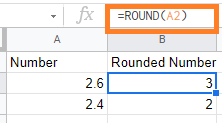In the above example,

• The decimal value 2.6 is converted to integer value 3. Since the right side number is greater than 5 the google sheets ROUND function is rounding up.
• The decimal value 2.4 is converted to integer value 2. Since the right side number is lesser than 5 the google sheets ROUND function is rounding down.

The round formula in google sheets will round up or round down the decimal value to the nearest integer value.

### Rounding Numbers to Nearest Decimal Point in Google Sheets

To round any decimal value to the nearest decimal point, we can use the ROUND function in Google sheets.

Below is the syntax,

=ROUND(value, places)

value – we should specify the decimal number which has to be round

places – if you want to round to one decimal place, you’d enter the value “1” for places

Example:

• =ROUND(9.134, 1) – This will give you 9.1
• =ROUND(9.136, 2) – This will give you 9.14
• =ROUND(111.123, -1) – This will give you 110
• =ROUND(253.12, -2) – This will give you 300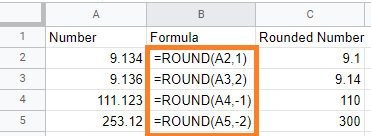In the above example,

• The decimal value (9.134, 1) is rounded to 9.1. Since we are specifying the places with “1” only one value is returned.
• If the second number is greater than 5 then the value is increased, i.e., = ROUND(9.165,1) gives you 9.2
• If the second number is lesser than 5, then the value is returned as the same, i.e., =ROUND(9.134,1) gives you 9.1
• The decimal value (9.136, 2) is rounded to 9.14. Since the third value is greater than 5, the second number is rounded up.
• The decimal value (111.123, -1) gives you 110. Here Google sheets round function will round up to 10s, 100s, and so on if you use negative place value. Since the 111.123 decimal number is round down to the number 110
• The decimal value (253.12,-2)  gives you 300. Since the nearest value to the (253.12, -2) number is 300. If we have the number (243.12, -2)  then the results would be 200

### ROUNDUP Function in Google Sheets

ROUNDUP function in google sheets helps you to round up the number as specified in places. This ROUNDUP function is similar to the ROUND function in google sheets.

Syntax:

=ROUNDUP(value, places)

• value – we should specify the decimal number which has to be rounded up
• places -we should specify the decimal place value that has to round up

Example:

• =ROUNDUP(2.623, 0) – This will give you 3
• =ROUNDUP(1.134, 1) – This will give you 1.2
• =ROUNDUP(3.906, 2) – This will give you 3.91
• =ROUNDUP(111.568, -1) – This will give you 120
• =ROUNDUP(253.555, -2) – This will give you 300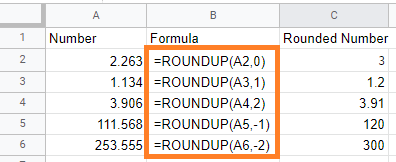In the above example,

• The decimal value (2.263, 0) is rounded to 3. Since the right side number is greater than 5, it is rounded to the next greater integer number.
• The decimal value(1.134, 1) is rounded up to 1.2. Here, the value of the place is mentioned as “1”. It returns the first right number on the right side.
• If the next number is greater than 5 then it will be rounded up
• If the next number is lesser than 5 then it will be rounded down
• The decimal value(3.906, 2) is rounded up to 3.91. Here, the right side of the third number is greater than 5 so the second number is rounded up.
• The decimal value (111.568, -1) is rounded up to 120. The negative value of the places will be rounded up to the nearest 10’s or 100’s.
• The decimal value of (253.555, -2) is rounded up to 300. Since the left side number is greater than 250 it is rounded up to 300. If it is less than 250 i.e., (241.555, -2) it is rounded up to 200.

ROUNDDOWN function in google sheets helps you to round down the number as specified in places. This ROUNDDOWN function is similar to the ROUND function in google sheets.

Syntax:

=ROUNDDOWN(value, places)

• value – we should specify the decimal number which has to be rounded down
• places -we should specify the decimal place value that has to round down

Example:

• =ROUNDDOWN(2.623, 0) – This will give you 2
• =ROUNDDOWN(1.134, 1) – This will give you 1.1
• =ROUNDUDOWN(3.906, 2) – This will give you 3.9
• =ROUNDDOWN(111.568, -1) – This will give you 110
• =ROUNDDOWN(253.555, -2) – This will give you 200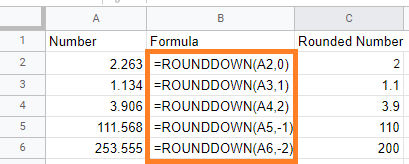In the above example,

• The decimal value (2.263, 0) is rounded down to 2. Even if the right side number is greater than 5, the ROUNDDOWN function in google sheets will round down.
• The decimal value(1.134, 1) is rounded down to 1.1. Here, the value of the place is mentioned as “1”. It returns the first right number on the right side.
• The decimal value(3.906, 2) is rounded down to 3.9. Here, the right side of the third number is 0, hence it is ignored.
• The decimal value (111.568, -1) is rounded down to 110. The negative value of the places will be rounded up to the nearest 10’s or 100’s.
• The decimal value of (253.555, -2) is rounded down to 200. Here, the nearest 100’s to the given number is 200.

### MROUND Function in Google Sheets

Using the multiple round functions in Google Sheets, you can take your rounding capabilities to the next level. Instead of a decimal position, these functions round to the nearest multiple of a given factor.

Syntax:

=MROUND(value,factor)

MROUND function in google sheets will round to the factor’s nearest multiple.

Example:

• =MROUND(14, 5) – This will give 15.
• =MROUND(12, 5) – This will give 10.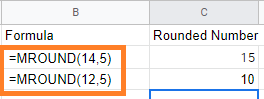In the above example,

The MROUND function in google sheets will round to the nearest multiple of 5.

• The number 14 is nearest to 15 in 5 multiple tables.
• The number 12 is nearest to 10 in 5 multiple tables.

### Round Number with the Formula in Google Sheets

Google Sheets allows you to return a rounded result by using ROUND as a wrapper.

For example, suppose we want to add the values in cells “A2” and “B2,” but we want the result rounded to the nearest whole number.

To accomplish this, we can use the formula “=SUM(A2:B2)” to calculate the value of “A2” plus “B2.” We can enclose that formula within =ROUND as follows:

=ROUND(SUM(4.44, 5.71) – This will give you 10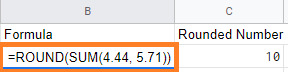Here, 4.44 is rounded to 4, and 5.71 is rounded to 6, hence we get the 10 as the result.

Google Sheets’ built-in rounding functions are an extremely versatile set of tools to use on your workbooks.

## How to Insert a Pivot Table in Google Sheets? (Create/Edit/Customize Pivot Tables)

Spreadsheets are an excellent tool for working with data. They assist you in organizing your data so that you can gain useful information and insight from it. Google Sheets includes the Pivot table feature, which makes data analysis even more powerful.

## What are Pivot Tables in Google Sheets?

Pivot tables aid in the summarization of data, the discovery of patterns, and the reorganization of information. You can either add pivot tables based on Google Sheets suggestions or create them manually. You can add and move data, add a filter, drill down to see details about a calculation, group data, and more after you create a pivot table.

Follow the steps as listed below to add a pivot table with the help of Google Sheets suggestion:

• Step 2: Now at the bottom of the Google Spreadsheet, click on the “Explore” button. Alternatively, you can also use the Keyboard shortcut (Alt+Shift+X) to enable the Explore button.
• Step 3: The explore menu will open up on the screen. Now click on the Pivot Tables shown up in the window.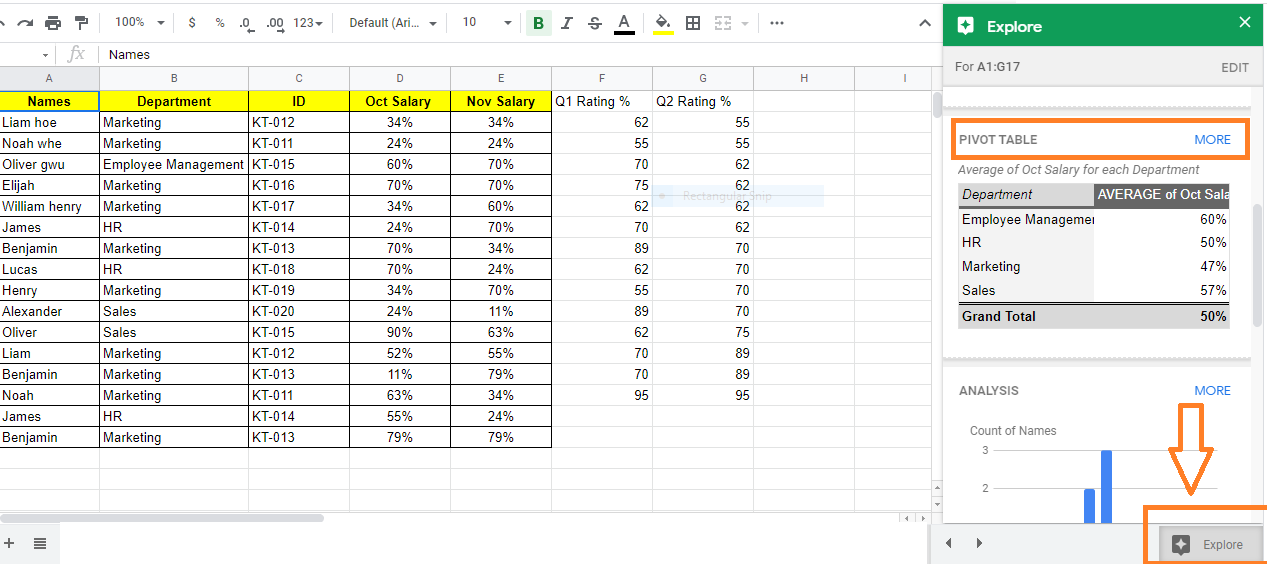• Step 4: Now the preview link of the Pivot table will be shown on the screen. Click on “Insert Pivot Table“.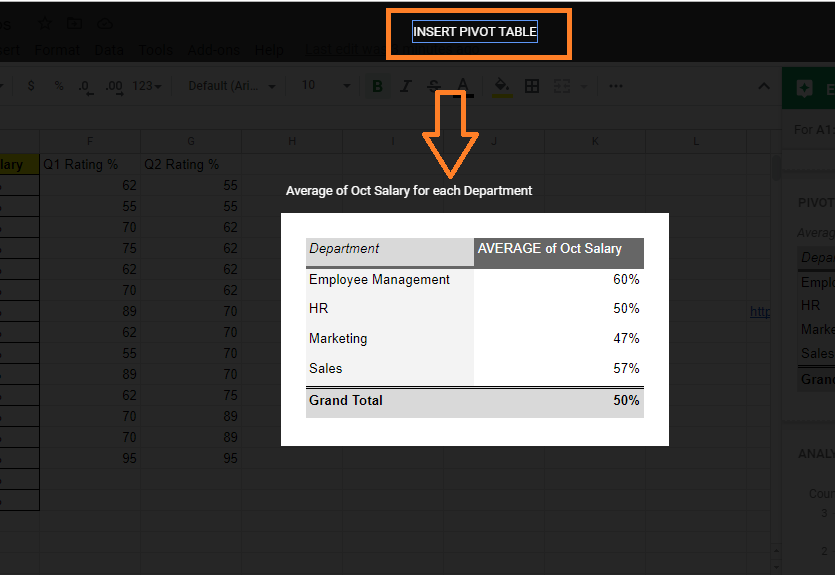• Step 5: That’s it, the new Pivot table is added to the sheet.

Note: If a Pivot table is not applicable to your data, the pivot table wouldn’t be generated on the sheet. In order to insert the Pivot table in Google Sheets, the data you use should be organized into columns, with a header for each column.

### How to Create Pivot Table Manually in Google Sheets?

Follow the steps as listed below to create a Pivot table manually in Google Sheets:

• Step 2: Click on the Data Tab and select Pivot table from the drop-down menu.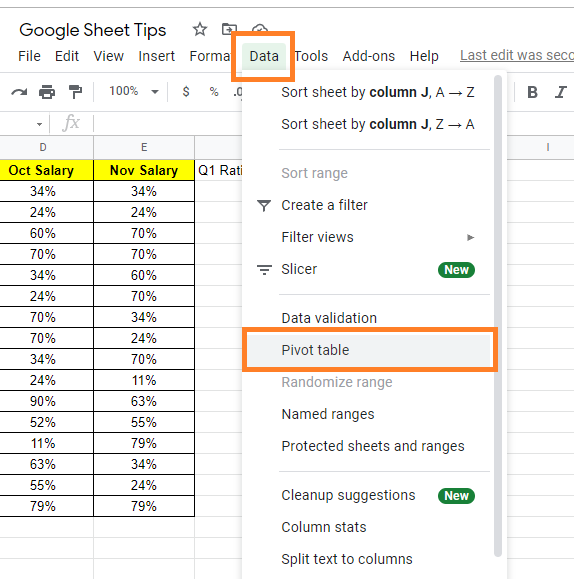• Step 3: Now a window will appear on the screen. In this window choose Data Range.
• Step 4: In the same window, there will be two options named “Insert To“. Here select New Sheet to create a Pivot table in the new sheet or select Existing Sheet to create the pivot table in the existing sheet.
• Step 5: Now click on the “Create” button.• Step 6: Enter the cell range where the Pivot table should be created. Now the Pivot table editor will open towards the right side of the screen. With the help of the Pivot table editor, you can customize your table.

### How to Edit or Customize Pivot Table in Google Sheets?

Firstly in order to edit the Pivot table, click anywhere on the table. The Pivot table editor window will open towards the right side of the screen. Now you will be provided with a list of the following options with the help of which you can customize the Pivot tables in Google Sheets.

To Add Data: In the Pivot table editor, you will see 4 options namely rows, columns, filter, values. Just click on the Add button below each section to add the data.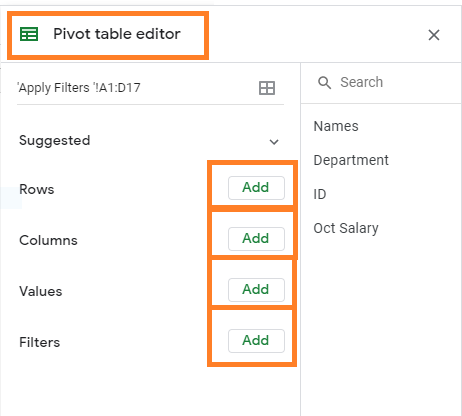To Change Row/Column Name: Double click the row or the column to change the name.

To Change Sort Order/Column: Click the Down arrow under Rows or Columns. Select the option or item under Order or Sort by. This will sort the data.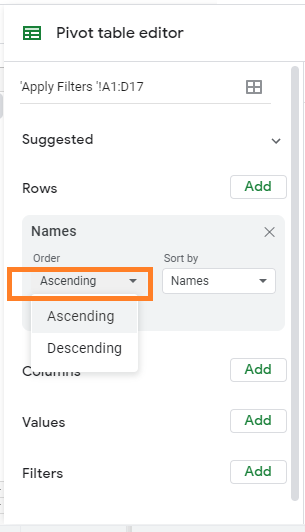To Change the Data Range: Select the data range and enter the new range.

To Delete Data: To delete the data, click on the “X” icon as shown below. This will delete the data which was created earlier.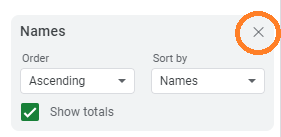To Show Totals: Check the Show Totals box under Rows or Columns.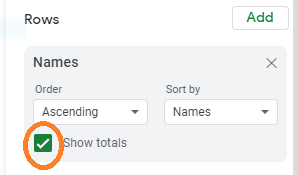To Show Values as Percentages: Under Values, click the Down arrow, then select a percentage option under Show as.

### How to Hide Data With Filter in Pivot Table?

We can hide data with filters in the Pivot table by following the steps as listed below:

• Step 1: Click on the Pivot table and the Pivot table editor will open towards the right side of the screen.
• Step 2: Now move the Filter section.
• Step 3: Click on the “Add” button. Select the column or row which needs to be included in the Filter.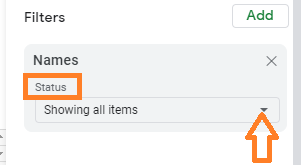• Step 4: Now under the “Status” section, select the list of rows or columns which need to be hidden.
• Step 5: Click on the “Ok” button.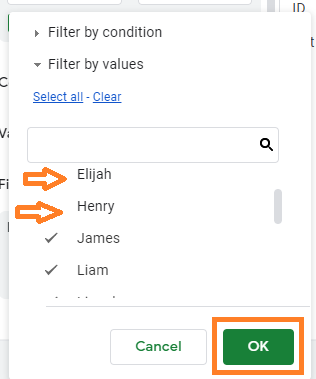The selected row or column would be hidden.

Google Sheets are very helpful when it comes to organizing the data in one place. With the help of various Google Sheet functions, we can create amazing templates such as Scorecard, Annual Budget, To-Do Lists, Attendance, Leave Tracker, and so on.

In this article, let’s understand how to create a Leave Tracker Template with the help of Google Sheet tips provided on this page. Also if you like the leave tracker template which has been created by us, you can make a copy of it and use it for further reference.

## Google Sheets Leave Tracker Template

Here is the snapshot of my sample leave tracker.## How to Create Leave Tracker Sheets in Google Sheets?

Follow the steps as listed below to create a Leave Tracker Sheet in Google Sheets:

• Step 2: Create a Sheet tab and name it as Attendance Key.
• Step 3: In the Attendance Key tab, Enter the number of leave types you are offering to your employees or students. In the above example, I have created 4 types of attendance keys namely Paid Leave, Loss of Pay, CompOff, Maternity Leave.

Refer to the image below.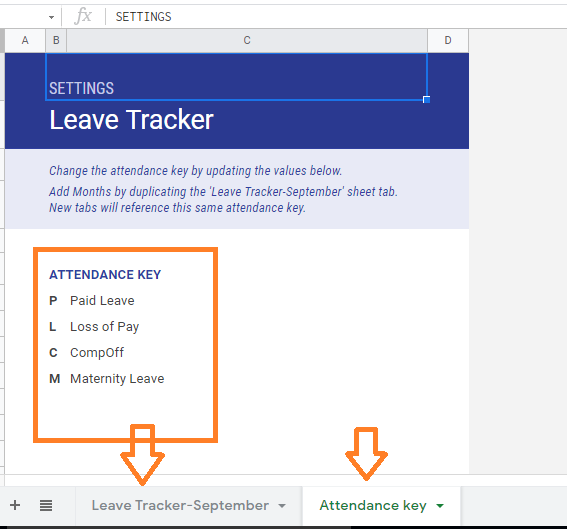• Step 4: Now create another tab and name it as Leave Tracker Sheet. In this example, I am creating September Leave Tracker Sheet.
• Step 5: In cells A and B, we have listed out the employee ID. You can also modify this cell with the employee name or other unique ID of the employee.
• Step 6: Now in cell C2, enter the date from which you tracking the leaves in the format of MM/DD/YYYY. In our case, I am tracking the leave of employees from 4th September 2021.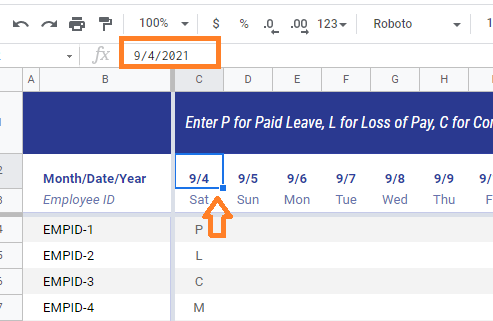• Step 7: Now move to cell C3 and enter the formula as “=C2“. This will generate the day of the dates entered in cell C2.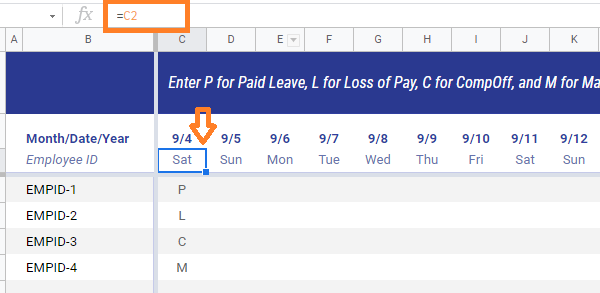• Step 8: Select Cell C2 & C3 and drag the fill handle. This will auto populate the date and dates till the column you drag the fill handle
• Step 9: Once the sufficient date and days are filled out, Add four more columns.
• Step 10: Now, enter the following formulas in each column respectively. So that it will fetch the data entered in Attendance Key Tab
• =‘Attendance key’!\$C7 (C7 is Paid Leave)
• =‘Attendance key’!\$C8 (C8 is Loss of Pay)
• =‘Attendance key’!\$C9 (C9 is CompOff)
• =‘Attendance key’!\$C10 (C10 is Maternity Leave)
• In my case, I have created 4 additional columns from the cell W. Thus the four columns I have created are W, X, Y, Z.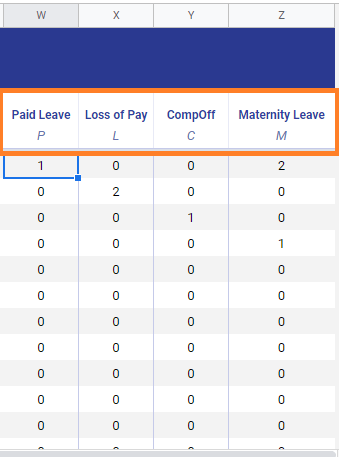• Step 11: Now move to the Paid leave cell and enter the formula as “=countif(\$C4:\$V4,W\$3)
• Step 12: Next to the Loss of Pay cell, enter the formula as “=countif(\$C4:\$V4,X\$3)“. In CompOff and Maternity Leave columns, enter the formulas “=countif(\$C4:\$V4,Y\$3)” and “=countif(\$C4:\$V4,Z\$3)” respectively.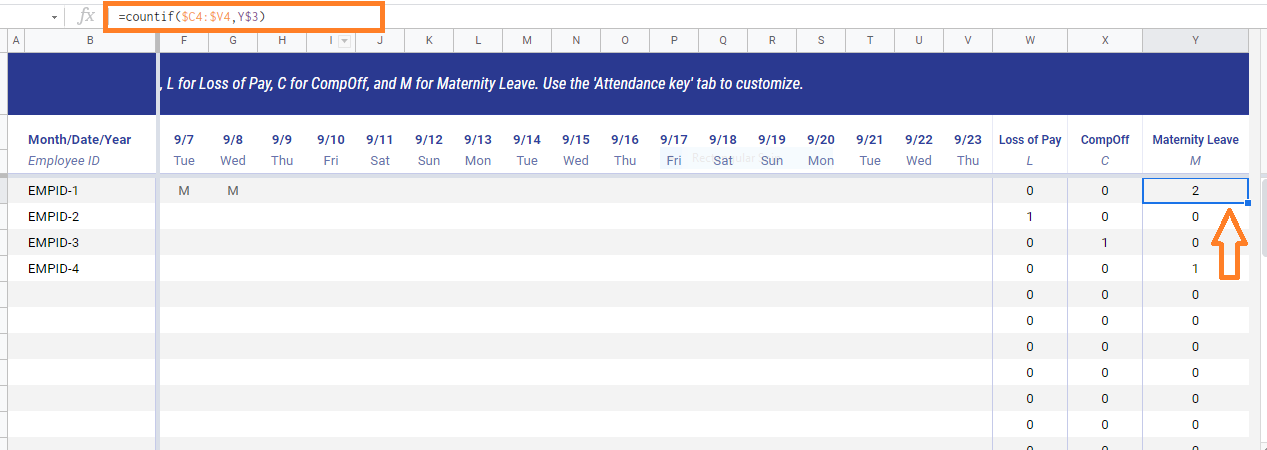• Step 13: Now move to the cell C1 and enter the formula as “=iferror(concatenate(” Enter “, ‘Attendance key’!\$B\$7, ” for “,‘Attendance key’!\$C\$7, “, “, ‘Attendance key’!\$B\$8, ” for “, ‘Attendance key’!\$C\$8, “, “, ‘Attendance key’!\$B\$9, ” for “, ‘Attendance key’!\$C\$9, “, and “, ‘Attendance key’!\$B\$10, ” for “, ‘Attendance key’!\$C\$10, “. Use the ‘Attendance key’ tab to customize. “))“. You will the results as shown below: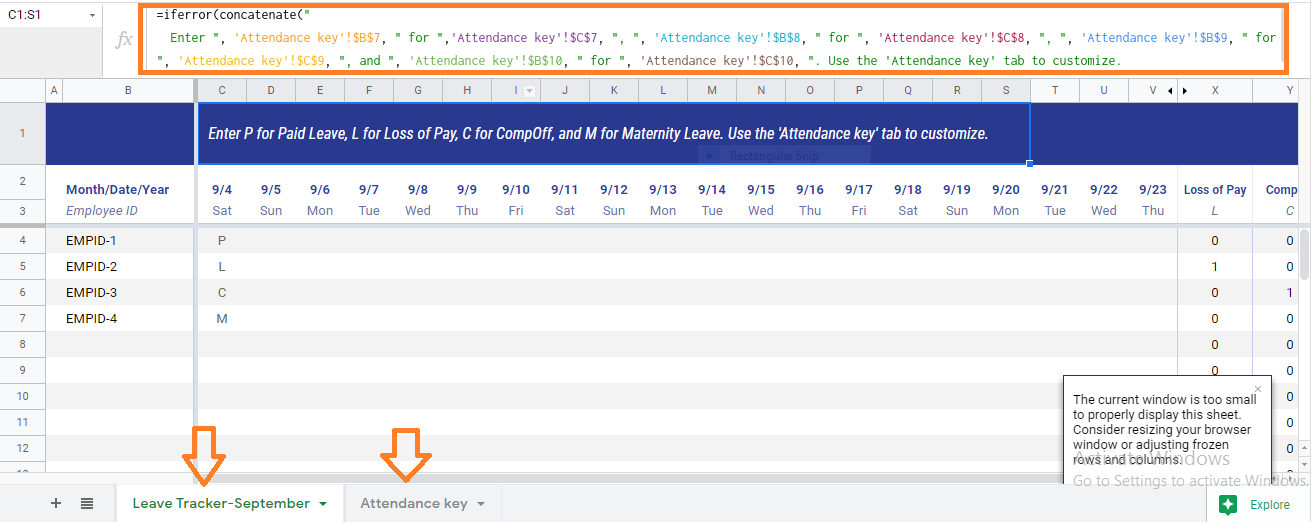• Step 14: The leave tracker is created. Now all you have to do is enter the type of leave the employee has taken. Based on the type of leave taken, the sheet will update the number of leave and the type of leave that employee has taken.

In this example, I have mentioned that employee 1 has taken 2 maternity leaves. Thus the maternity leave tab shows the total number of maternity leaves taken by the employee.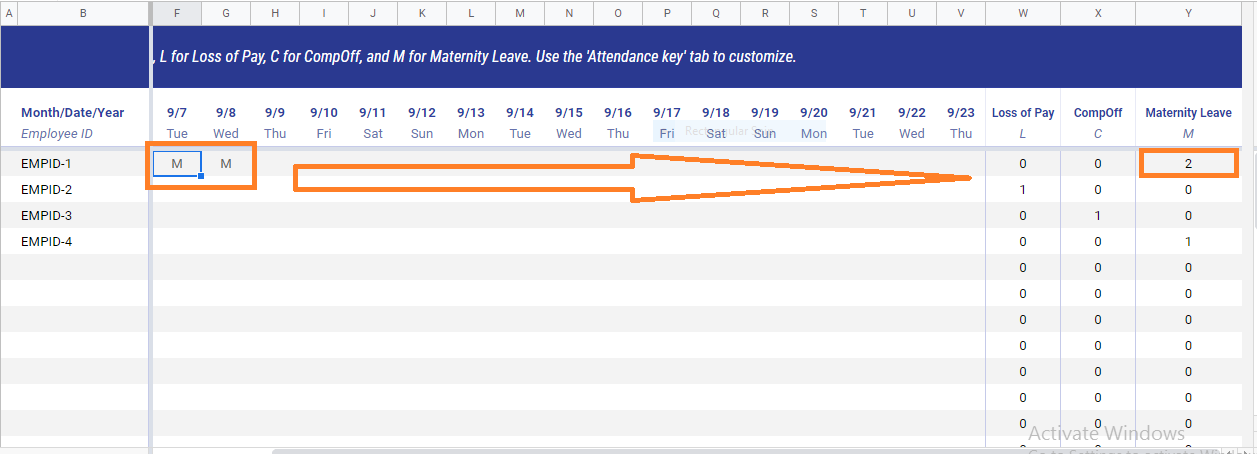Click on the following link to make a copy of the Leave Tracker Sheet.

In order to create a month-wise leave tracker sheet, add M=months by duplicating the ‘Leave Tracker-September’ sheet tab. New tabs will reference this same attendance key.

## How to Hide Columns from Certain Users in Google Sheets (3 Easy Methods)

When you are working with Google Sheets which needs to be shared with multiple users, you might fall under a situation where you might want to hide columns from certain users which is impossible. However, there are few hacks with the help of which you can hide the columns in Google Sheets from certain users. In this article, let us understand how to hide columns/rows from certain users with the help of Google Sheet hacks provided on this page. Read on to find more.

## Hiding Columns in Google Sheets

Consider the following dataset where you want to hide columns B and C.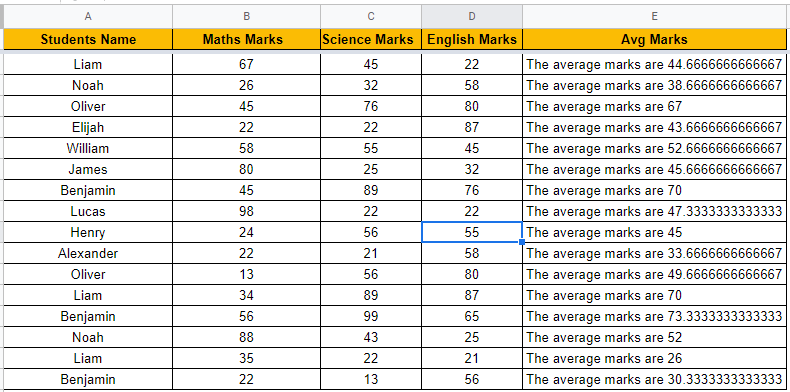We can easily hide the columns in Google Sheets with the help of the steps provided below:

• Step 1: Select the column headers which you would like to hide.
• Step 2: Now Right-click anywhere on the screen.
• Step 3: The context menu will open. Now select “Hide Column” from the drop-down menu.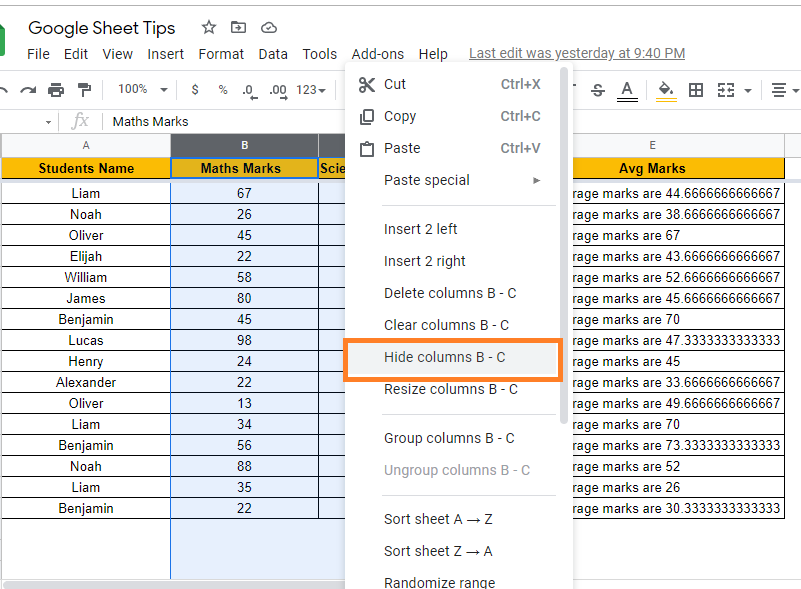• Step 4: The selected columns will be hidden now as shown below.Note: This method is used to hide columns temporarily. If any user clicks on the hidden arrow, then the hidden columns will be unhidden. However, if you use this method while printing the worksheet, the hidden columns will not be printed.

Know how to Unhide Columns.

Since this is a temporary hiding method of columns, let’s use a protected range method to hide columns from certain users in Google Sheets.

### Use Protect Range Feature to Hide Columns from Certain Users

With the help of Protect Range feature in Google Sheets, we can restrict certain users to edit the selected range of cells, be it column or row. Let’s understand how to hide columns with the help of the Protected range feature in Google Sheets by following the steps listed below:

• Step 1: Select the headers of the columns which you would like to hide and Right-click anywhere on the sheet.
• Step 2: Now the context menu will open on the screen. Here select “Protect Range” from the drop-down menu.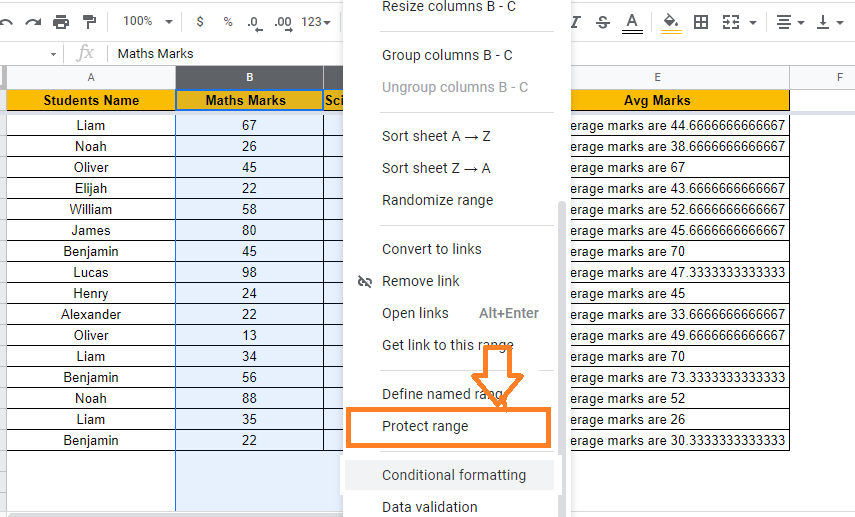• Step 3: Protect Sheet and ranges sidebar will open on the screen. Now enter the cell range in the Range window.
• Step 4: Now click on the “Set Permissions” button.
• Step 5: Now the Range Editing Permission window will open on the screen. Now check the “Restrict who can edit this range” box.
• Step 6: Under “Choose who can edit” you will be provided with the list of users who have edit access to the sheet. You can simply uncheck their email ids. Doing so will protect the selected columns by shared users.
• Step 7: Click the “Done” button.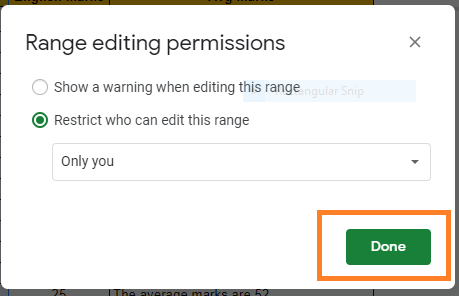• Step 8: Now again move to the column headers and right-click anywhere on the cell.
• Step 9: Select “Hide Columns” from the context menu and this will hide the selected columns.

Note: You will see that the columns have been hidden, but users who don’t have edit access will not be able to unhide them, so they won’t be able to see them or make any edits.

Nevertheless, there’s a snag in the works: There is still a chance that they will be able to see the content of this column. This is because, if the shared persons make a copy of this sheet, then they can easily see the unhidden columns and make changes in the copy sheet.

### Using IMPORTRANGE Function to Hide Columns from Certain Users

IMPORTRANGE Function in Google Sheets helps us to access data from one workbook to another sheet. Thus we can use the IMPORTRANGE function to hide columns from certain users in Google Sheets. Let us understand how to do this with the help of the steps given below:

• Step 1: Firstly, create a private Google Sheet which contains the columns that need to be hidden from certain users.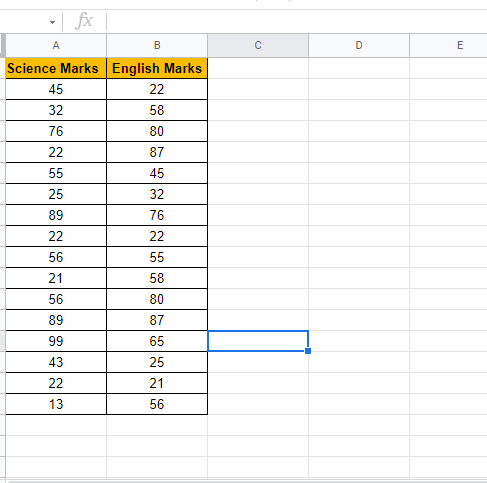• Step 2: Now share this private Google Sheet to the selected users to whom you wish to make the columns accessible.
• Step 3: Then move to the Sheet which is been shared with others. Here go the column and use the IMPORTRANGE function to import the columns.
• Step 4: The formula to IMPORT the columns in our case is “=IMPORTRANGE(“url”,”Sheet1!B1:B9)“.
• Step 5: Press the “Enter” button.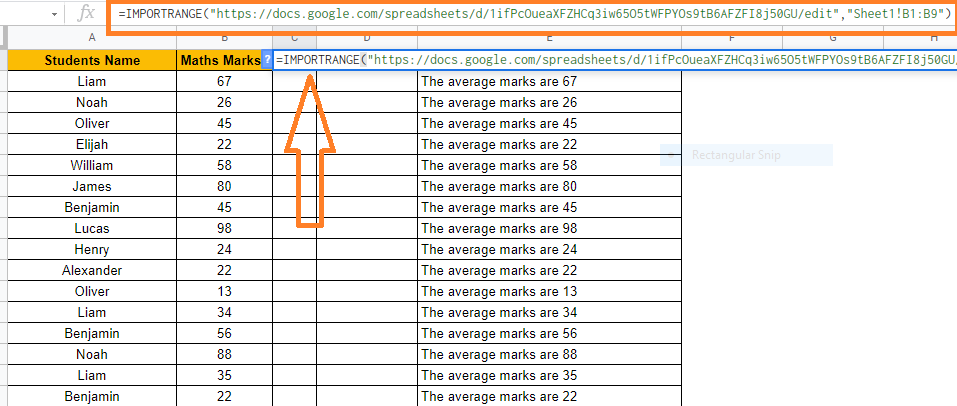• Step 6: Now the columns will be imported. Then protect the columns with the help of steps provided in the previous section.
• Step 7: Then Right-click the columns which need to be hidden and select “Hide Column” from the context menu.
• Step 8: Now the columns will be hidden.

How does this work?

In this case, all of the columns will be copied to the new sheet once more. The user can still see that there are some columns that are hidden.

When they try to unhide the columns, they will encounter a #REF! error. They will see a message that says, “You don’t have permission to access that sheet,” when they hover over the cell containing the error message.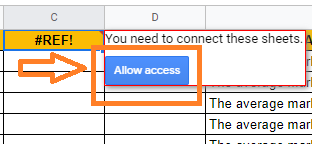This is due to the IMPORTRANGE function attempting to access a file to which the user does not have access. If you want to make changes to the data in the hidden columns, you must do so in the private file. Because the IMPORTRANGE function is dynamic, any changes you make will be automatically reflected in your shared file.

## How to Delete Every Other Row in Google Sheets (Using Filter & Formula)

If you want to delete every other row in Google Sheets manually, it is a time-consuming process. In order to save your time, you can use ISEVEN or MOD function, with the help of which you can delete every other row, Nth row, third row, and so on.

## Delete Every Other Row using in Google Sheets using Filter Icon

Follow the steps as listed below to delete every other row in Goole Sheets using the Filter icon:

• Step 1: Select the columns where you would like to delete every other in Google Sheets.
• Step 2: Apply the Filter using “Filter Icon” from the toolbar.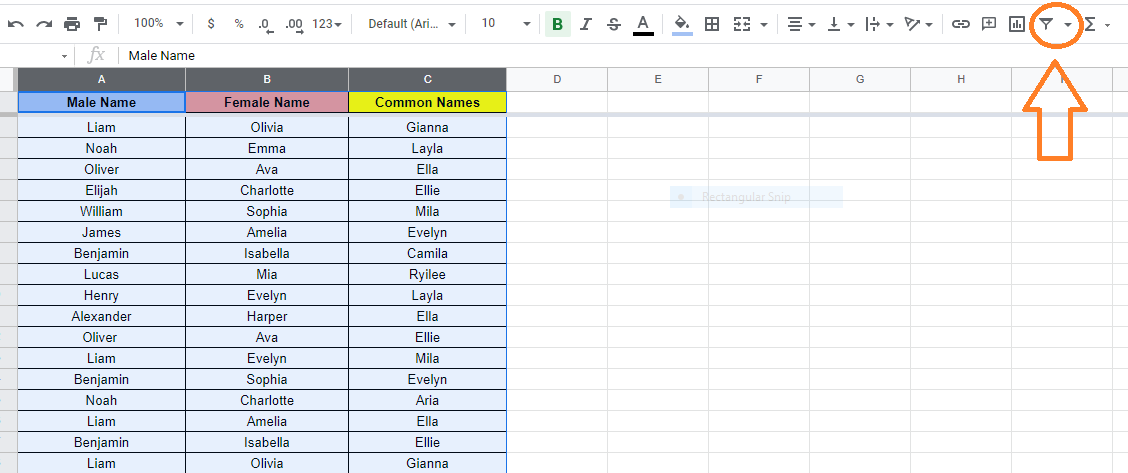• Step 3: Now click on the Filter icon in the column header and select “Filter by Condition” from the drop-down menu.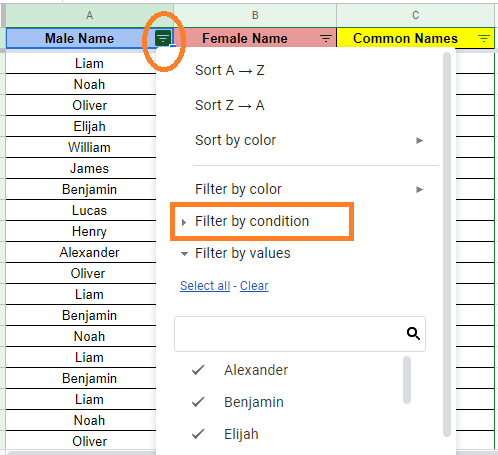• Step 4: Select “Custom Formula” in the empty field.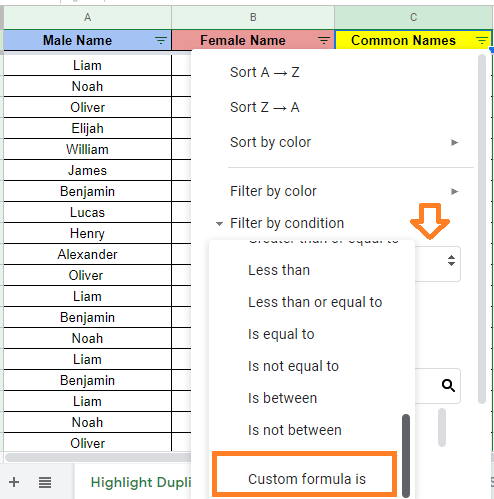• Step 5: Now enter the formula as “=ISEVEN(row(A1))“.
• Step 6: Click on the “Ok” button. You will see the results.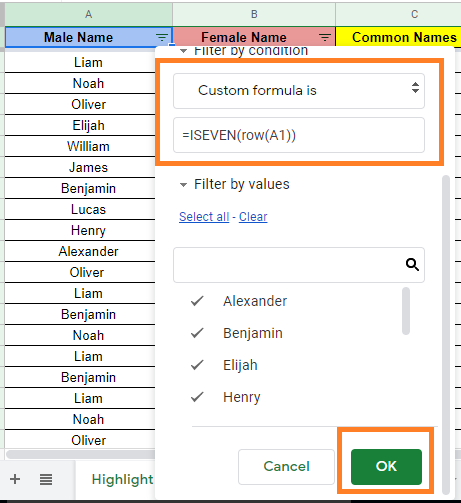• Step 7: Now select all of the fields on the worksheet (Ctrl+A on a PC, CMD+A on a Mac), “Copy” the values (Ctrl+C on a PC, CMA+C on a Mac), and then click the “+” icon to make a new worksheet/page.
• Step 8: Paste the visible contents from the previous worksheet/page into the new worksheet/page (Ctrl+V for PC, CMD+V for Mac) by clicking on cell A1 on the new worksheet/page. Every other row in the worksheet has now been erased.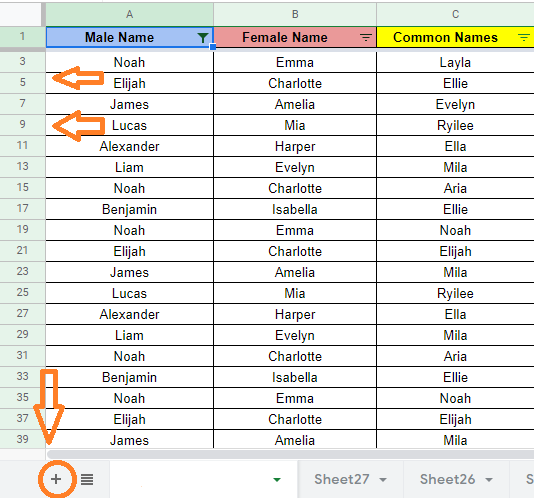### How to Delete Nth Row in Google Sheets?

Deleting every other row in Google Sheets is quite easy. But if you want to delete the 3rd row, 5th row, 6th row, and so on, then you can use the MOD function. Though the MOD function is a bit tricky, it deletes the Nth row as per the requirement.

Let’s understand how to do this by following the steps listed below:

• Step 1: Select the columns where you want to delete the Nth row.
• Step 2: Now apply the filter to the selected columns using the Filter icon.
• Step 3: Once the filter is applied, click on the Filter icon in the column header.
• Step 4: Select Filter by condition and select “Custom Formula” from the drop-down menu.• Step 5: Now enter the formula =MOD((row(A1) – row (\$A\$1)-3),4) in the empty field.
• Step 6: Press the “Enter” button and you will see the results.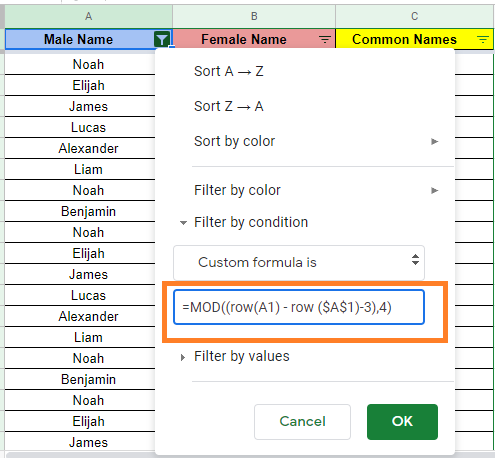Now copy-paste the results to another sheet in the spreadsheet to delete the hidden rows.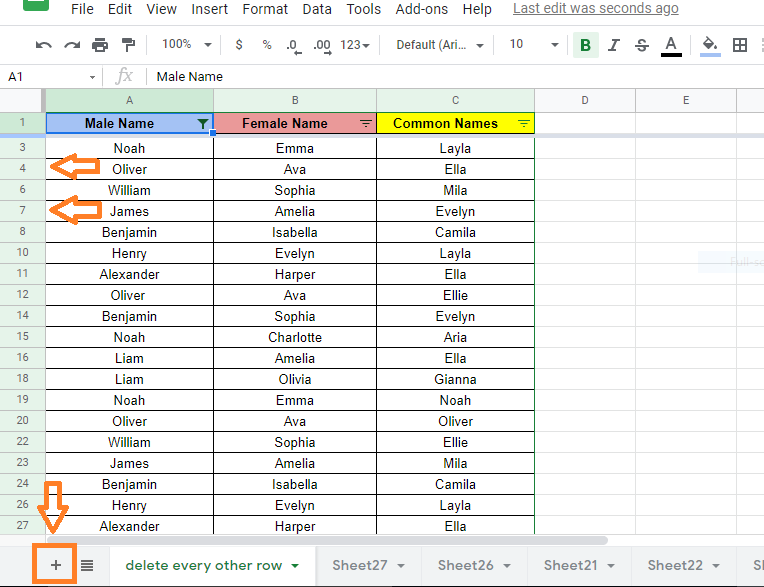### Explanation of MOD Formula in Google Sheets

MOD Function Syntax =MOD((row(A1) – row (\$A\$1)-{Nth -1}),{Nth})

• MOD(dividend, divisor): This is the modulus operator; we’re using it to hide or remove the cell if the division operation gives a remainder of “0.”
• Row: The value of a cell’s row is returned by ROW(cell reference). This is how we indicate that we’re looking at rows rather than individual cells.
• Offset: Determines which row begins the hiding/deleting period. Calculate the offset by subtracting 1 from your Nth factor to begin on the Nth cell after the header ( if N is 7, the offset is 6).
• The first row AFTER the header is hidden by -0.
• After the header, -1 starts obscuring the second row.
• After the header, -2 starts hiding the third row.
• After the header, -3 starts hiding the fourth row.

This pattern repeats itself until it shares the same value as N. After a certain point, you can’t use this formula to start hiding rows.

For example, if you want to delete every third row, use =MOD((row(A1) – row (\$A\$1)-2),3) and similarly for every tenth row, use the following formula =MOD((row(A1) – row (\$A\$1)-9),10.